Page top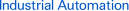# FAQ04321 of Programmable Relays FAQ

FAQ No. FAQ04321

Question

ZEN Programmable Relays: Can I use AC 2-wire sensors?

﻿

AC 2-wire sensors cannot be connected directly to the AC input of the ZEN. To connect a 2-wire sensor, insert a relay between the sensor and the ZEN or connect external bleeder resistance.

Example: Connecting to a CPU Unit or Expansion I/O Unit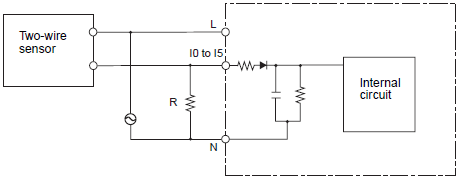a) Use a resistance that satisfies both of the following conditions.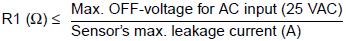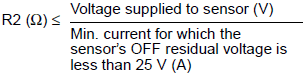b) Because of heat generation, use a resistor with at least the following wattage.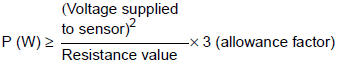Note:

Calculating the Bleeder Resistance when Connecting an OMRON E2E-X10Y 2-Wire AC Sensor
This calculations in this example are based on an input voltage of 85 to 110 V AC.
The following values are from the Sensor datasheet.
The Sensor's maximum leakage current is 1.7 mA.
The minimum current when the Sensor's OFF residual voltage is 25 V or less (i.e., the OFF voltage for the EN) is 5 mA, as shown in the residual voltage characteristic graph.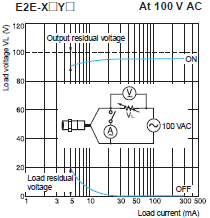From the formulas given above,

R1 ≤ 25 V AC/1.7 mA = 14.7 kΩ
R2 ≤ 85 V AC/5 mA = 17 kΩ

The bleeder resistor must thus be 14 kΩ

The Sensor output current in this case would be 100 VAC/14 kΩ, or 7 mA. This satisfies the Sensor's control output range of 5 to 300 mA.

The bleeder resistor capacity is calculated as shown below and must be 3 W.

P ≥ (110 V AC2)/14 kΩ × 3 = 2.59 W

## Recommended Products

###K3HB-X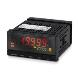A Process Indicator Ideal for Discriminating and Displaying Measurements for Voltage/Current Signals

###E5CN-H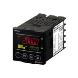A New High-performance Controller: High Resolution, High Speed, and High Input Accuracy. Logic Operations and Preventive Maintenance Function.

###H5CZ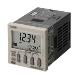Easy to Use and Easy to Read.